# SAT Math Multiple Choice Question 75: Answer and Explanation

### Test Information

Question: 75

15. Which of the following is equivalent to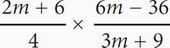?

• A.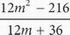• B.• C.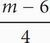• D. m - 6

D Whenever there are variables in the question and answers, think Plugging In. If m = 2, the expression becomes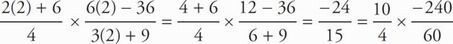= - 4. Plug 2 in for m in the answers to see which answer equals the target number of -4. Choice (A) becomes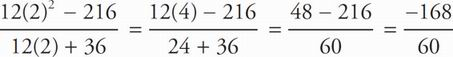= - 2.8. This does not match the target number, so eliminate (A). Choice (B) becomes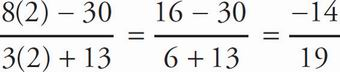. Eliminate (B). Choice (C) becomes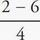=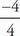= -1. Eliminate (C). Choice (D) becomes m - 6 = 2 - 6 = -4. The correct answer is (D).